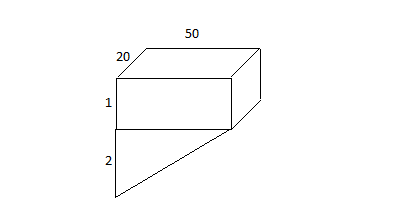# A swimming pool is 50m long and 20m wide. Its depth decreases linearly along the length from 3m...

## Question:

A swimming pool is 50m long and 20m wide. Its depth decreases linearly along the length from 3m to 1m. It is initially empty and is filled at a rate of {eq}1\text{m}^3 {/eq}/min.

(a) How fast is the water level rising 640 min after the filling begins?

(b) How many minutes will it take to fill the pool?

## Volumes:

This problem requires solving for the volume of a figure. If there is no direct way to solve for the volume of a figure, it would be more convenient to divide the figure into parts wherein the volume of the parts can easily be solved, then adding the volume of the parts gives the volume of the whole figure.

## Answer and Explanation:A) Note that since the volume of the triangular part of the pool is {eq}\frac{1}{2}(2m)(50m)(20m)=1000m^3 {/eq} (area of the triangle times the...

See full answer below.

Become a Study.com member to unlock this answer! Create your account